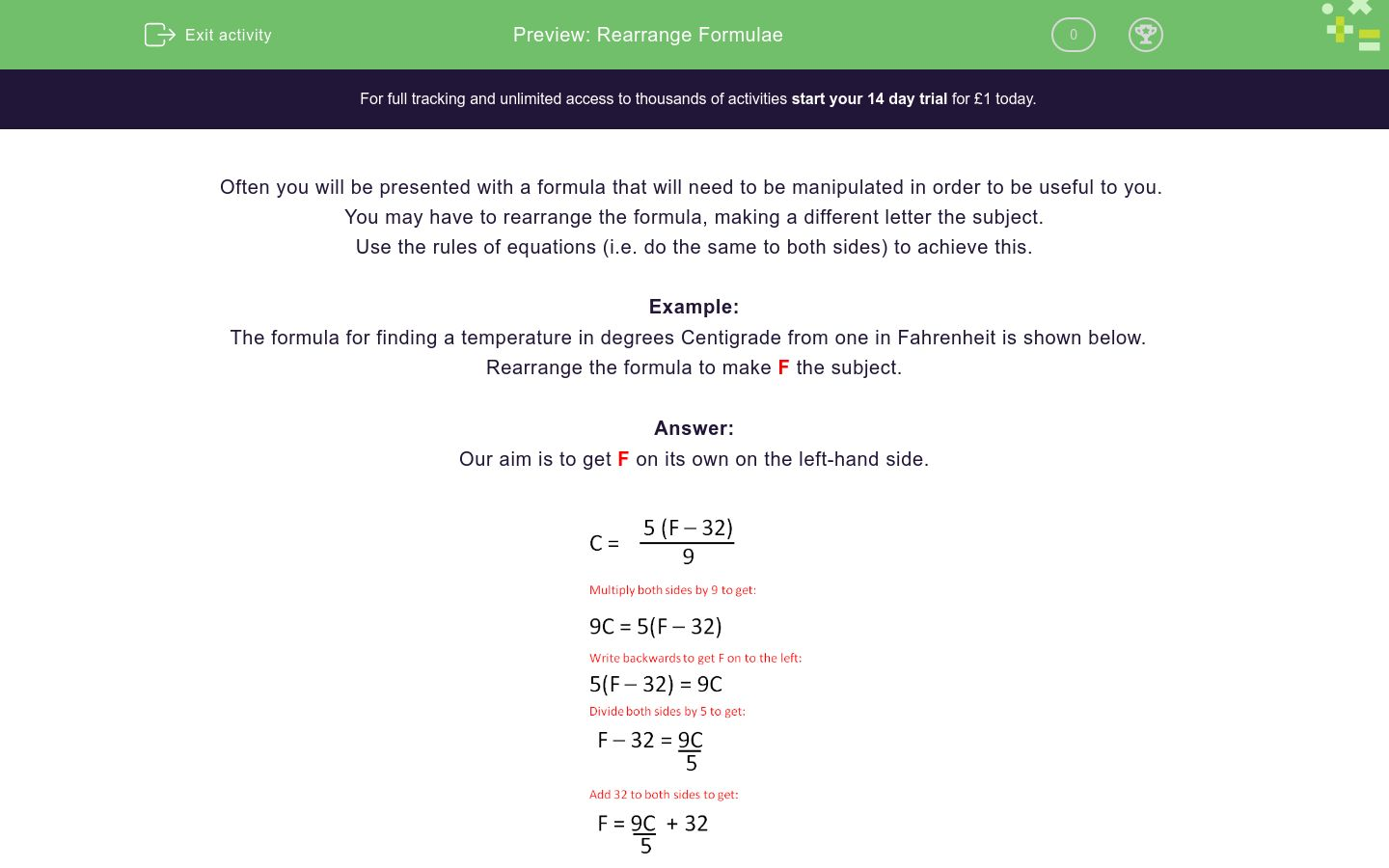# Rearranging Formulae

In this worksheet, students rearrange formulae to make the given letter the subject of the formula.Key stage:  KS 4

Curriculum topic:  Algebra

Difficulty level:### QUESTION 1 of 10

Sometimes you are given a formula that will need to be manipulated to be useful to you.  You may have to rearrange the formula to make a different letter the subject.

Use the rules of equations (i.e. do the same to both sides) to achieve this.

Example

The formula for finding a temperature in degrees Centigrade from one in Fahrenheit is shown below.  Rearrange the formula to make F the subject.

Our aim is to get F on its own on the left hand side.Rearrange the following formula to make w the subject.

v = lwh

l = vwh

w = vlh

w = v/lh

w = v/l/h

Rearrange the following formula to make u the subject.

v = u + at

u = v + at

u = v - at

u = v/at

u = vat

Rearrange the following formula to make a the subject.

v = u + at

a = v + ut

a = v - ut

a = (v - u)t

a = (v - u)/t

Rearrange the following formula to make c2 the subject.

a2 = b2 + c2

c² = a² + b²

c² = a² - b²

c² = b² - a²

c² = a² - b²

Rearrange the following formula to make c the subject.

a2 = b2 + c2

c² = a² + b²

c = √a² - b²

c = √(b² - a²)

c = √(a² - b²)

Rearrange the following formula to make h the subject.

A = ½bh

h = ½Ab

h = 2A/b

h = ½A/b

h = 2Ab

Rearrange the following formula to make h the subject.

P = 2(b + h)

h = ½Pb

h = 2P/b

h = ½P - b

h = 2P - b

Rearrange the following formula to make u the subject.

s = ut + ½at2

u = (s - ½at²)/t

u = v - at

u = st - 2at²

u = 2t² - ½at

Rearrange the following formula to make a the subject.

s = ut + ½at2

a = (s - ½at²)/t

a = sut²

a = st - 2ut²

a = 2(s - ut)/t²

Rearrange the following formula to make r the subject.

A = 4πr2

r = 4π/A

r = ½√π/A

r = √(A/4π)

r = √(4π/A)

• Question 1

Rearrange the following formula to make w the subject.

v = lwh

w = v/lh
EDDIE SAYS
Divide both sides by lh
• Question 2

Rearrange the following formula to make u the subject.

v = u + at

u = v - at
EDDIE SAYS
Subtract at from both sides
• Question 3

Rearrange the following formula to make a the subject.

v = u + at

a = (v - u)/t
EDDIE SAYS
Subtract u from both sides and then divide by t
• Question 4

Rearrange the following formula to make c2 the subject.

a2 = b2 + c2

c² = a² - b²
EDDIE SAYS
Subtract b² from both sides
• Question 5

Rearrange the following formula to make c the subject.

a2 = b2 + c2

c = √(a² - b²)
EDDIE SAYS
Subtract b² from both sides and then square root both sides
• Question 6

Rearrange the following formula to make h the subject.

A = ½bh

h = 2A/b
EDDIE SAYS
Double both sides and then divide by b
• Question 7

Rearrange the following formula to make h the subject.

P = 2(b + h)

h = ½P - b
EDDIE SAYS
Halve both sides and then subtract b
• Question 8

Rearrange the following formula to make u the subject.

s = ut + ½at2

u = (s - ½at²)/t
EDDIE SAYS
Subtract ½at² from both sides and divide by t
• Question 9

Rearrange the following formula to make a the subject.

s = ut + ½at2

a = 2(s - ut)/t²
EDDIE SAYS
Subtract ut from both sides, double both sides and divide by t²
• Question 10

Rearrange the following formula to make r the subject.

A = 4πr2

r = √(A/4π)
EDDIE SAYS
Divide both sides by 4π and square root
---- OR ----

Sign up for a £1 trial so you can track and measure your child's progress on this activity.

### What is EdPlace?

We're your National Curriculum aligned online education content provider helping each child succeed in English, maths and science from year 1 to GCSE. With an EdPlace account you’ll be able to track and measure progress, helping each child achieve their best. We build confidence and attainment by personalising each child’s learning at a level that suits them.